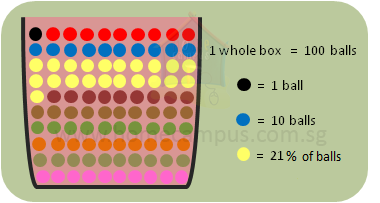## Introduction to Percentage

Practice Unlimited Questions

#### What is meant by percentage or percent?

We use percentage all the time in our day-to-day lives. Here are some examples:
1. At the supermarket: 2% milk
2. In the mall: 70% off the price
3. A saying: Give it your 100%
The word percent is made up of 2 words - per and cent. Let's first see what cent is.
\$1  =  100¢
1¢  = 1/100th of a \$

So,
percent  =  1/100th  =  1 part out of 100 parts

Similarly,
5 percent  =  5 parts out of 100 parts
Note:
We use % to denote percent. So,
1%  =  1 percent
5%  =  5 percent

#### 1.  The basket below contains 100 balls of different colours. a)   What percentage of balls in the basket is black? b)   What is the percentage of blue balls in the basket? c)   How many yellow balls are there?Total number of balls in the basket  =  100

a)  Black ball  =  1 out of 100  =  1%
So, 1% of balls in the basket is black.

b)  Blue balls  =  10 out of 100  =  10%
So, the basket contains 10% blue balls.

c)  Yellow balls  =  21%  =  21 out of 100
So, there are 21 yellow balls.